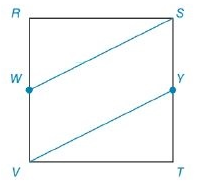Chapter 8.2, Problem 55EElementary Geometry For College St...

7th Edition
Alexander + 2 others
ISBN: 9781337614085

Solutions

Chapter
SectionElementary Geometry For College St...

7th Edition
Alexander + 2 others
ISBN: 9781337614085
Textbook Problem

Each side of square R S T V has length 8. Point W lies on V R - and point Y lies on T S - in such a way to form parallelogram V W S Y , which has an area of 16   u n i t s 2 . Find the length of V W - .To determine

To Find:

The length of VW- in the square provided.

Explanation

Formula Used:

1. Pythagorean theorem for the right angle triangle ABC for the hypotenuse AC,

AC2=AB2+BC2.

2. The area A of a square whose sides are each of length s is given by A=s2.

3. The area A of a parallelogram with a base of length b and with corresponding altitude of length h is given by A=bh.

4. Area of right triangle =12bh, where b is the base and h is the height of the triangle.

Calculation:

It is given that Each side of square RSTV has length 8 with point W lies on VR- and point Y lies on TS- in such a way to form parallelogram VWSY.

Also given that area of the parallelogram VWSY is 16 units2.

Since VWSY is a parallelogram, opposite sides are equal in measure. That is, VW = SY.

RW = RV-VW=TS-SY=TY

Since RW = TY, the triangle SRW and the triangle VTY must be same

Still sussing out bartleby?

Check out a sample textbook solution.

See a sample solution

The Solution to Your Study Problems

Bartleby provides explanations to thousands of textbook problems written by our experts, many with advanced degrees!

Get Started

Simplify: 3656

Elementary Technical Mathematics

Find the derivative. Simplify where possible. 33. h(x) = sinh(x2)

Single Variable Calculus: Early Transcendentals, Volume I

For y=12gt2 (where g is a constant), dydt=. a) 14g2 b) 12g c) gt d) gt2

Study Guide for Stewart's Single Variable Calculus: Early Transcendentals, 8th

In Exercises 1926, pivot the system about the circled element. [013241562|434]

Finite Mathematics for the Managerial, Life, and Social Sciences

The directrix of the conic given by is:

Study Guide for Stewart's Multivariable Calculus, 8th